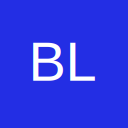# FD11 command reference. New in nachi robots

• Hello, I started to work with nachi robots, after many years working with ABB, Fanuc, and Kuka.
I have a basic manual, but I looking for Command Reference manual, for FD11 controller. Because I want to learn the instructions set.
For example Move-X instruction, I want to know which means al parameters in this instruction.

/BlueIcaro

• Do you have the robot with you? If yes, there's a help button there...

MOVEX A=1, AC=0, SM=0, M1J, P, (0,90,0,0,-90,0), R=100.0, H=1, MS, CONF=0000

MOVEX: Command

A= Accuracy

AC= Acceleration

SM= Smoothness

M1J= Mechanism number and also set the type of information in the brackets M1E for encoder, M1J for joint angles, M1X for xyzrpy

P= Interpolation P for joint, L for linear, C1 for circle 1, C2 for circle 2

() information that was previously set with Mechanism number, also possible to move POSE number and POSITION variable

R= Rate in %, S in mm/s, T in time, D in deg/s

H= Tool number

MS= Speed master

CONF= Configuration such as lefty/righty, above/below elbow flip/nonflip J5